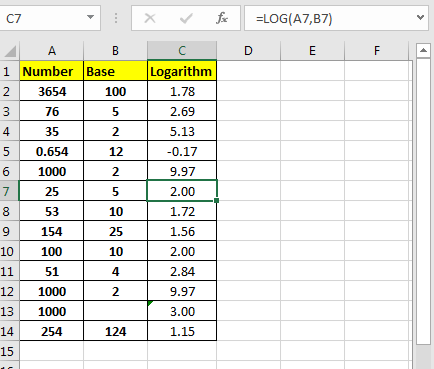The LOG function in Excel returns logarithm of any number with supplied base. If the base is not supplied, by default it is 10.Syntax of LOG Function

=LOG(number,[base])

Number: The number of which you want to calculate log in excel.
[base]: The base of the logarithm. By default, it is 10.
Excel LOG Function Example
To find the logarithm of 1000 with base 2 we can write this LOG Formula in Excel:

=LOG(1000,2)

To find log 1000 with base 10 we can write this LOG function in Excel:

=LOG(1000)

Note that we have not supplied the base argument here. Because by default it is 10.

Now if you know how to do log in excel. LOG function is used in the background to create a LOG scale graph.

Related Articles:

Excel LOG10 function

Popular Articles:

50 Excel Shortcuts to Increase Your Productivity

How to use the VLOOKUP Function in Excel

How to use the COUNTIF function in Excel 2016

How to Use SUMIF Function in Excel

Terms and Conditions of use

The applications/code on this site are distributed as is and without warranties or liability. In no event shall the owner of the copyrights, or the authors of the applications/code be liable for any loss of profit, any problems or any damage resulting from the use or evaluation of the applications/code.